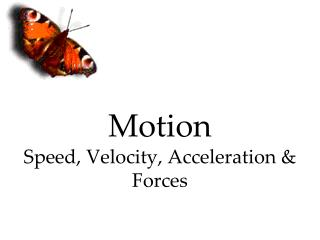# Motion Speed, Velocity, Acceleration & Forces - PowerPoint PPT PresentationDownload PresentationMotion Speed, Velocity, Acceleration & Forces

Loading in 2 Seconds...

Motion Speed, Velocity, Acceleration & ForcesDownload Presentation## Motion Speed, Velocity, Acceleration & Forces

- - - - - - - - - - - - - - - - - - - - - - - - - - - E N D - - - - - - - - - - - - - - - - - - - - - - - - - - -
##### Presentation Transcript

1. Motion Speed, Velocity, Acceleration & Forces

2. Frame of Reference Object or point from which motion is determined

3. What is Motion? Motion: A change in position of an object compared to a reference point Motion involves all of the following:

4. What is motion? • If you are standing in one place, and your friend walks by you, are you moving relative to your friend? • Is your friend moving relative to you? • Is either of you moving relative to the earth?

5. Answer: • You are moving relative to your friend, and your friend is moving relative to you! • You (the Joker) are not moving relative to the earth, but your friend is. You are both moving relative to the sun!

6. What is motion? • If you and your friend are walking down the hall together at the same speed, in the same direction, are you moving relative to your friend? • Is your friend moving relative to you? • Are either of you moving relative to the earth?

7. Answer: • You are NOT moving relative to your friend, and your friend is NOT moving relative to you. You both are moving relative to the earth.

8. What is Motion? = distance time

9. Speed Example: A car travels 300km in 6 hours. What is the speed of the car?

10. Answer: • Speed = distance ÷ time • Speed = 300km ÷ 6 hours • Speed = 50km/hr

11. More practice • 1. How far can a plane travel if it flies 800km/hr for 9 hours? • 2. How long does it take a ship to go 500 km if it travels at a speed of 50km/hr?

12. Answers • 7200km/hr • 10 hours

13. What is Motion?

14. Velocity • Speed in a given direction. • What is the velocity of a boat that travels from Lancaster to Baltimore(10 miles) in 15 minutes?

15. Answer • Speed = distance ÷ time • Speed = 10 miles ÷ 15 minutes • Speed = 0.67 mi/min • Velocity = 0.67 mi/min NW

16. Acceleration • Change in velocity • Can be change in speed or direction

17. Acceleration = Vfinal – Vinitial • Time or • = ∆Velocity • Time

18. Acceleration problem • A roller coaster’s velocity at the top of a hill is 10m/s. Two seconds later it reaches the bottom of the hill with a velocity of 26m/s. What is the acceleration of the roller coaster?

19. ANSWER: a = 26m/s – 10m/s 2 s a = 16m/s 2s a = 8m/s/s or 8m/s2

20. What is a Force? FORCE = Any push or pull which causes something to move or change its speed or direction

21. What is a Force? Forces can be BALANCED or UNBALANCED • Balanced forces are equal in size and opposite in direction • UNbalanced forces are not equal in size and/or opposite in direction. If the forces on an object are UNBALANCED, we say a NET force results.

22. Determine the NET FORCE: